# Mathematics Test 9 - Limits And Continuity, Coordinate Geometry, Application Of Derivatives

## 25 Questions MCQ Test Mock Test Series for JEE Main & Advanced 2022 | Mathematics Test 9 - Limits And Continuity, Coordinate Geometry, Application Of Derivatives

Description
Attempt Mathematics Test 9 - Limits And Continuity, Coordinate Geometry, Application Of Derivatives | 25 questions in 60 minutes | Mock test for JEE preparation | Free important questions MCQ to study Mock Test Series for JEE Main & Advanced 2022 for JEE Exam | Download free PDF with solutions
QUESTION: 1

###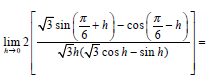Solution:

√3 sin(π/6+h) - cos( π/6+h)

2(√3/2 sin(π/6+h) - 1/2 cos( π/6+h))

2(cos(π/6) sin(π/6+h) - sin(π/6) cos( π/6+h))

2sin(π/6+h -π/6)

2 sin (h)

similarly in the denominator (this next step is not entirely necessary but I think it makes it eaiser)

(√3 cos(h) - sin(h))

2(cos(h+π/6))

lim h-->0 4 sin (h)/2√3 h cos(h+π/6)

(2/√3) (sin h / h)(1/cos h+π/6)

lim h-->0 (sin h / h) = 1

lim h-->0 (1/cos h+π/6) = sec(π/6) = 2/√3

(2/√3)2 = 4/3

QUESTION: 2

Solution:
QUESTION: 3

###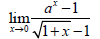Solution:
QUESTION: 4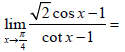Solution:
QUESTION: 5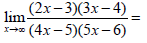Solution:

lim(x----∞) [x*x(2-3/x)(3-4/x)]/[x*x(4-5/x)(5-6/x)]
= (2*3)/(4*5)
= 6/20
= 3/10

QUESTION: 6

................................................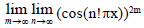equals

Solution:
QUESTION: 7

If f(a) = 2, f`(a) = 1, g(a) = –1, g`(a) = 2, then value of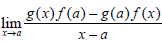Solution: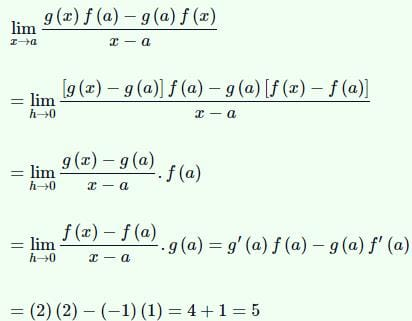QUESTION: 8

The vertices of a triangle ABC are (2,1),(5,2) and (3,4)respectively. The circumcentre is the point

Solution:
QUESTION: 9

If A and B are the points (–3,4) & (2,1). Then the co-ordinates of point C on AB produced such that AC = 2 BC are

Solution:
QUESTION: 10

The equation of the line passing through the intersection of x - √3 y + √3 - 1 = 0 and x y–2 = 0 and
making an angle of 150 with the first line is

Solution:
QUESTION: 11

If 2x2 + λxy + 2y2 +(λ - 4)x + 6y - 5 = 0is the equation of a circle, then its radius is

Solution: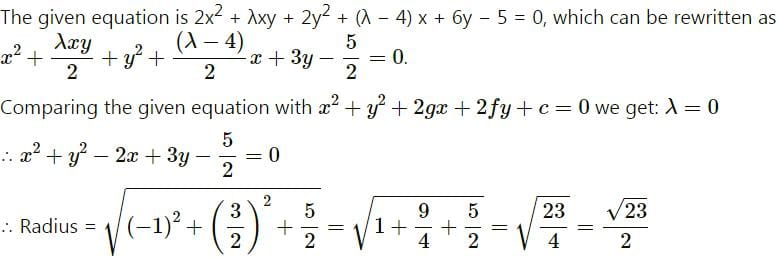QUESTION: 12

Equation of circles which pass through the points (1,–2) and (3,–4) and touch the x-axis is

Solution:
QUESTION: 13

The circle whose centre is on the x-axis and the line 4x–3y–12 = 0 and whose radius is the distance
between the line 4x–3y–32 = 0 and 4x–3y–12 = 0 has equation

Solution:
QUESTION: 14

Equation of the circle whose radius is 5 and which touches externally the circle x2 + y2 -2x - 4y - 20 = 0 at
the point (5,5) is

Solution:
QUESTION: 15

The number of integral values of  for λ which  x2 + y2 +λx + (1 - λ) y + 5 = 0is the equation of a
circle whose radius cannot exceed 5 is

Solution:
QUESTION: 16

The angle at which the circle x2 + y2 = 16 can be seen from the point (8,0) is

Solution:
QUESTION: 17

The slope of the tangent at the point (h,h) of the circle  x2 + y2  = a2is

Solution:
QUESTION: 18

If f (x) = [x sin p x] { where [x] denotes greatest integer function}, then f (x) is

Solution:
QUESTION: 19

The equation of the locus of the mid-points of the chords of the circle 4x2 + 4y2 - 12x + 4y +1 = 0that subtend an anlge of 2π / 3 at its centre is

Solution:
QUESTION: 20

The distance of the point (1,2) from the radical axis of the circles x2 + y2 +6x - 16 = 0 and x2 + y2 -2x + 6y = 0  is

Solution:
*Answer can only contain numeric values
QUESTION: 21

Let f(x) be a twice-differentiable function and f''(0) = 2. Then evaluate.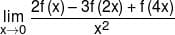Solution: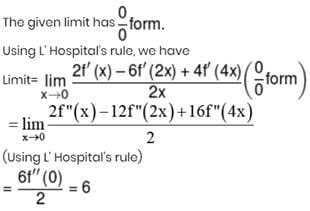*Answer can only contain numeric values
QUESTION: 22

The minimum value of  f(x) = ∣3 - x∣ +  ∣2 + x∣ + ∣5 - x∣  is:

Solution: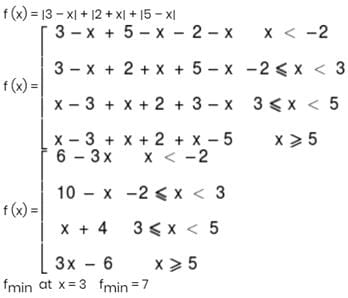*Answer can only contain numeric values
QUESTION: 23

If m is the slope of common tangent of y = x2 – x + 1 & y = x2 – 3x + 1, then |m| is

Solution:

Let point on curve y = x2 – x + 1 be
(x1,x2– x+ 1)
∴ Tangent is (y – x21 + x1 – 1) = (2x1 – 1)(x – x1
⇒ L1  : y = (2x1 – 1)x – x12 + 1
Let point on curve y = x– 3x +1 be (x2, x2– 3x+ 1)
∴ Tangent is  (y – x22 + 3x2 – 4) = (2x2 – 3)(x – x2)
⇒ L2 :  y = (2x2 – 3)x – x22 + 1
For common tangent, L1 & L2 are same.
⇒ –x12 + 1 = –x22 + 1
⇒ x1 = ±x2
& 2x1 – 1 = 2x2 – 3
x1 = x2 gives –1 = –3 (rejected)
x1 = –x2 gives x1 = – 1/2, x2 = 1/2
⇒ m = –2.

*Answer can only contain numeric values
QUESTION: 24

The volume of a tetrahedron DABC is 9 cubic units. If ∠ACB = π/6 and 2AD + AC + BC = 18, then  the length AD is

Solution: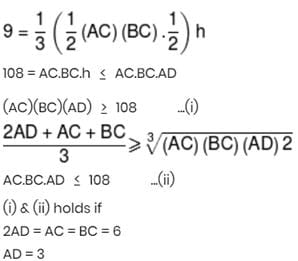*Answer can only contain numeric values
QUESTION: 25

If expression x + 1/x2 (x > 0) attains its minimum value at x = α, then α3 is

Solution: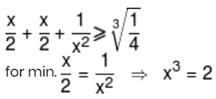Use Code STAYHOME200 and get INR 200 additional OFF Use Coupon Code